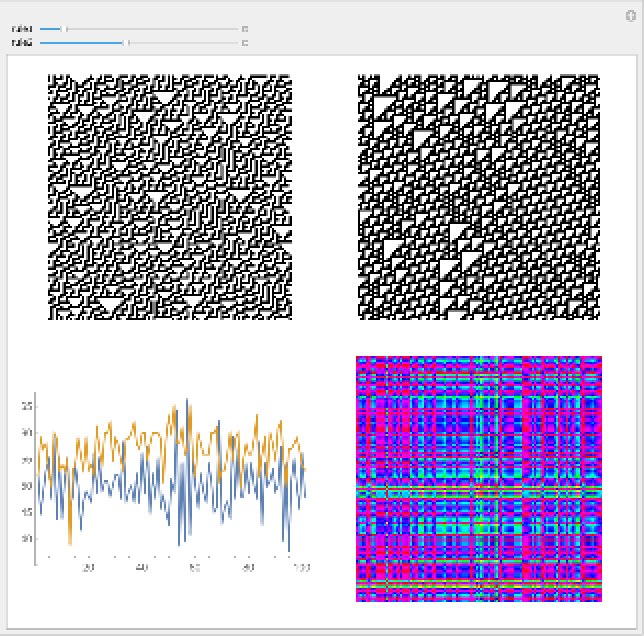#Function Repository Resource:

# CrossRecurrencePlot

Visualize the overlap of two discrete time series

Contributed by: Daniel de Souza Carvalho
 ResourceFunction["CrossRecurrencePlot"][ts1,ts2] views the cross recurrence plot of the given time series ts1 and ts2.

## Details and Options

ResourceFunction["CrossRecurrencePlot"] plots i on a horizontal axis and j on a vertical axis, where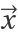is a phase space trajectory: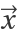(i)≈(j)
The recurrence plot is based on the function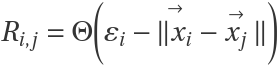where Θ is a unit step and ε a threshold
A recurrence plot is a matrix visualization where columns and rows correspond to a certain pair of times.
Setting the option "RecurrenceType""Global" replaces the function Θ used in the definition of Ri,j with the identity. The default setting of "RecurrenceType""Standard" preserves Θ.
The option “RecurrenceThreshold” allows the value of ε to be set.
CrossRecurrencePlot also takes all the options of ArrayPlot.

## Examples

### Basic Examples

View the cross recurrence plot of two random lists:

 In:=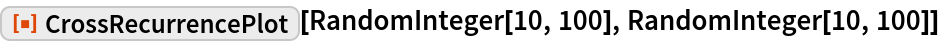Out=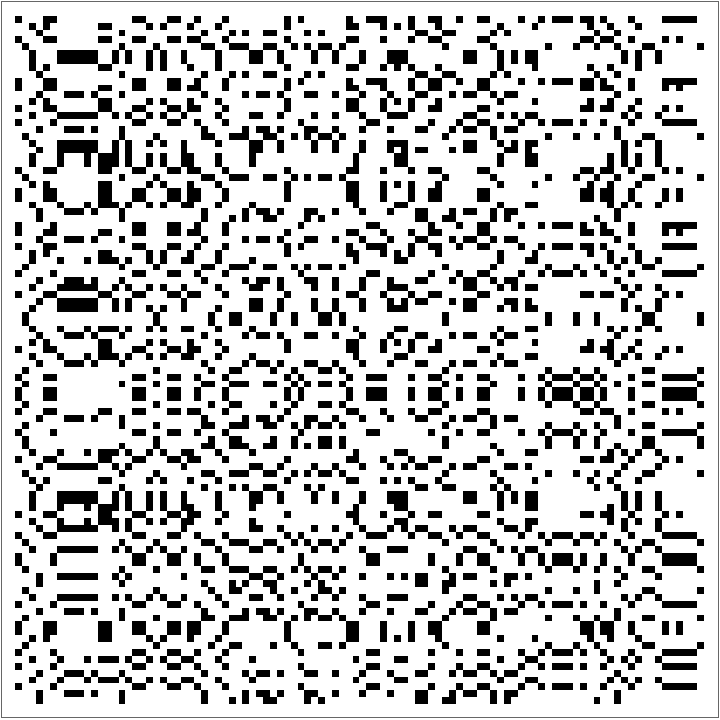View the cross recurrence plot of just one list of random integer values. Note the symmetry across the diagonal:

 In:=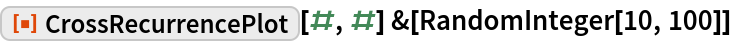Out=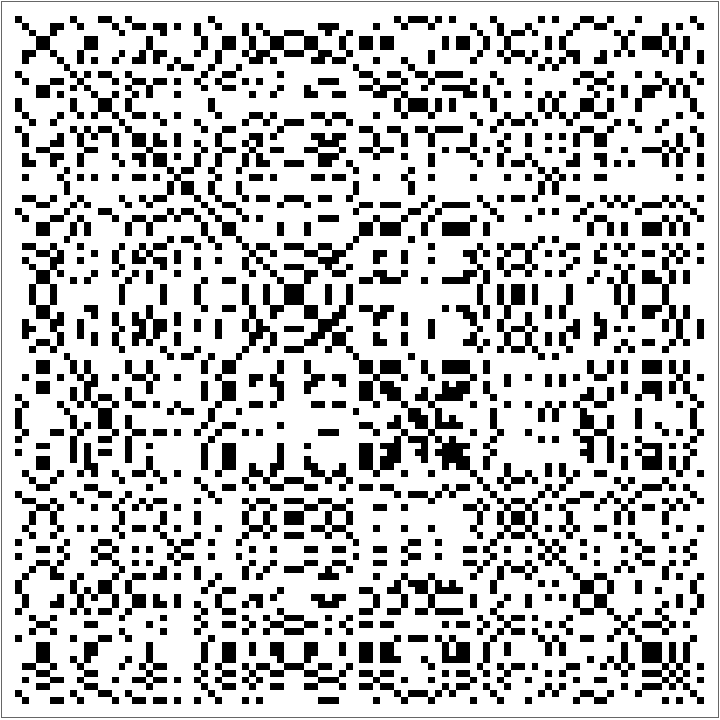Visualize the cross recurrence plot of the Sinc function with a recurrence type of "Global":

 In:=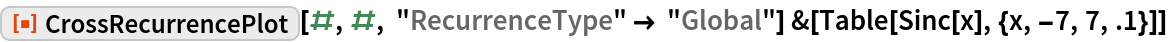Out=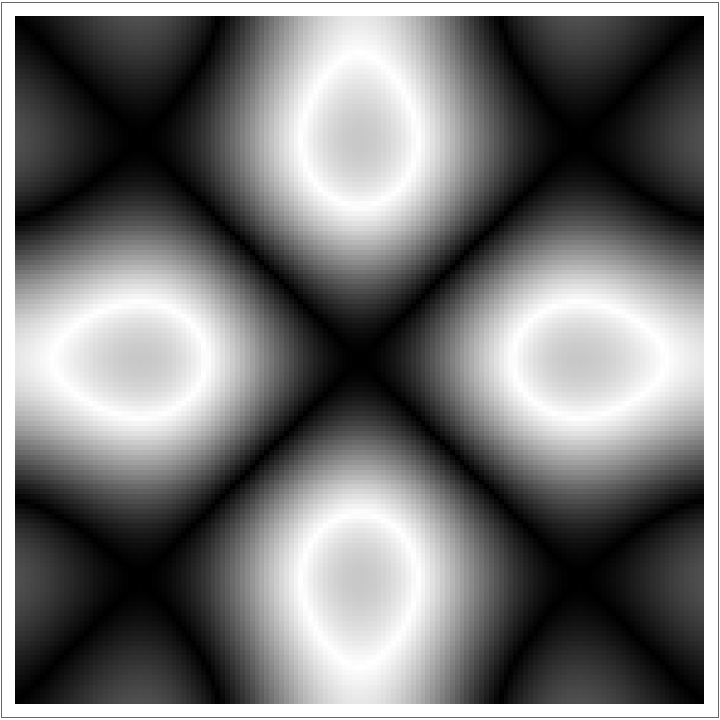View the cross recurrence plot of a range and a random list of integers:

 In:=Out=View a cross recurrent plot of equal ranges:

 In:=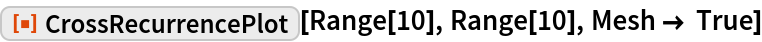Out=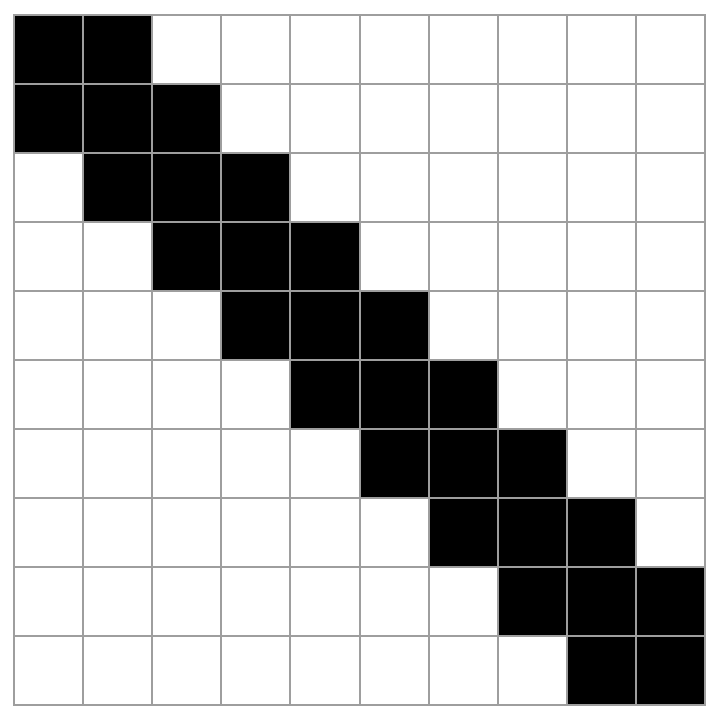View a cross recurrence plot of two different small lists:

 In:=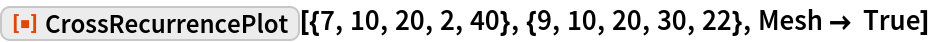Out=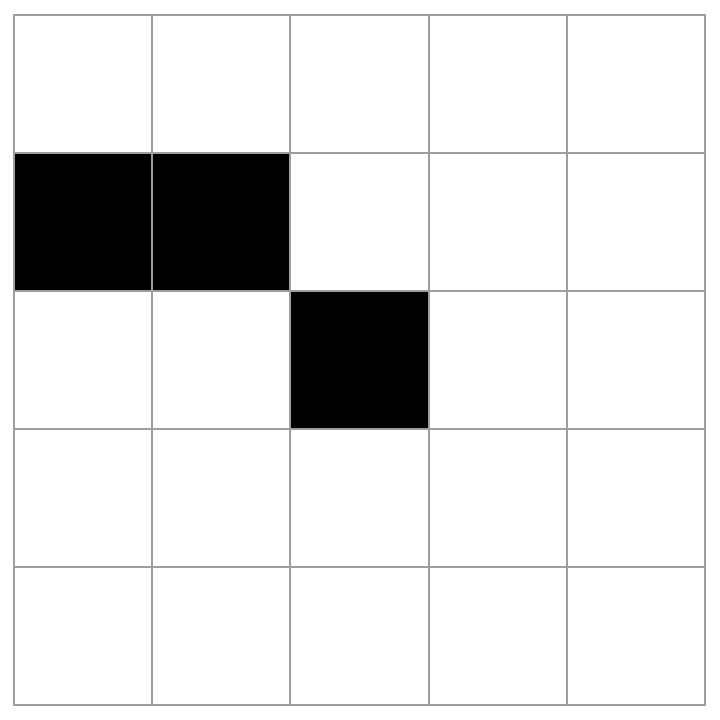View a cross recurrence plot of a range and a short list of integers:

 In:=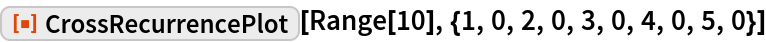Out=View the cross recurrence plot of discrete values of Sin and Cos:

 In:=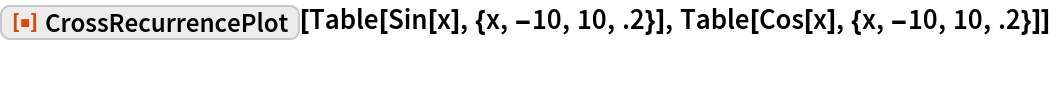Out=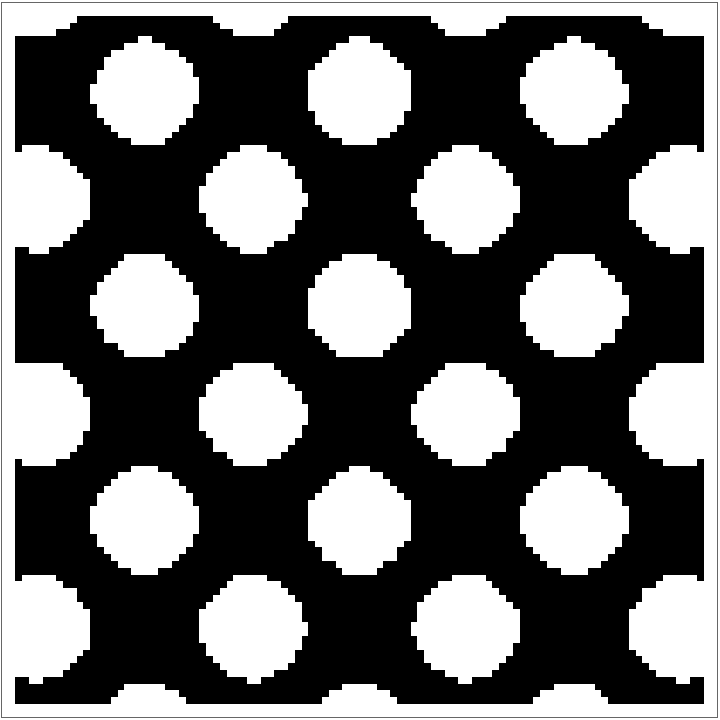View a cross recurrence plot of two lists of different sizes :

 In:=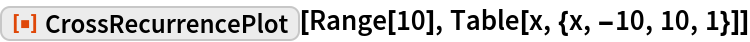Out=### Applications

We can analyse time series behaviour by cross recurrence plots. In this case we combine sine and cosine to create and visualize two different time series:

 In:=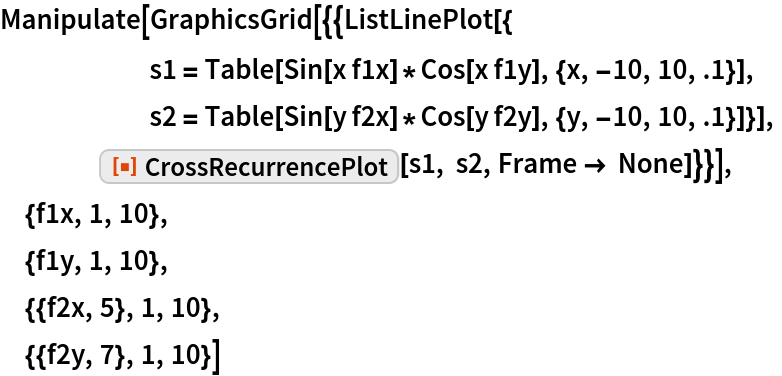Out=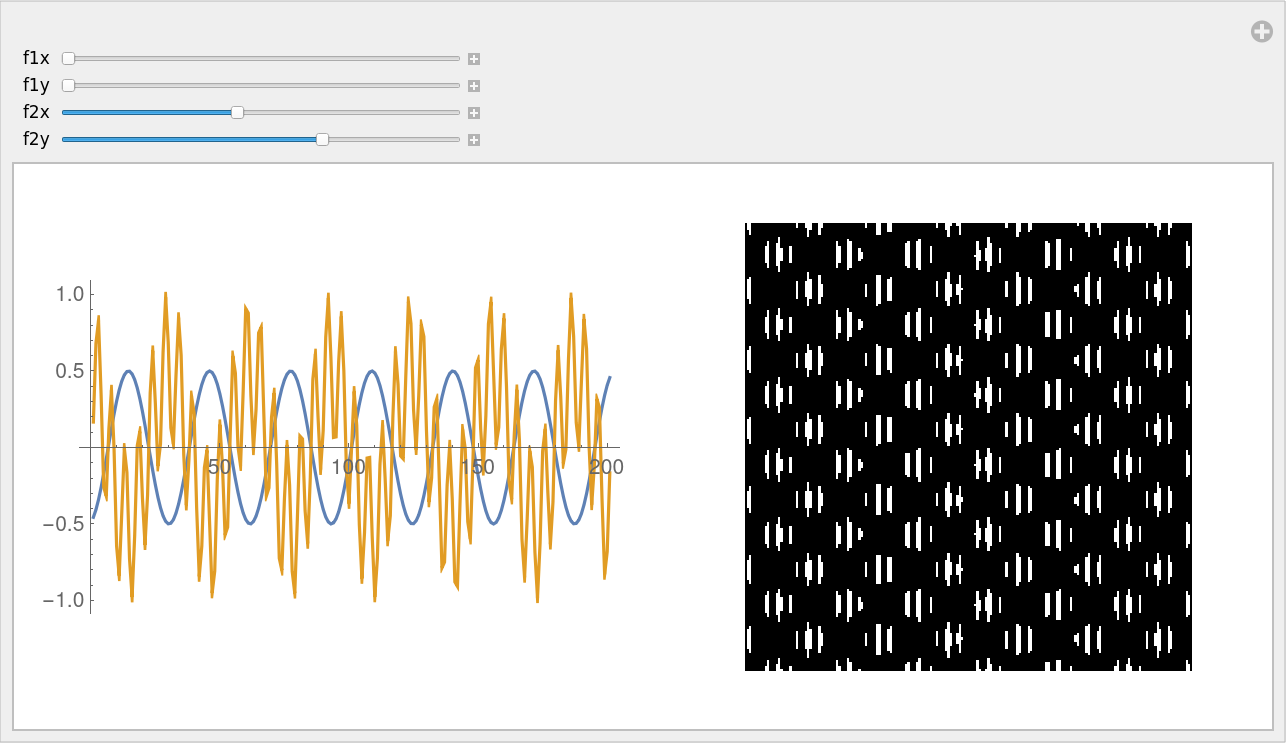To compare and analyse the dynamical behaviour of two elementary cellular automata rules we can plot them, count the total number of black cells by steps, and them show the global cross recurrence plot. Note that when we select the same rule for the two cellular automata, we see diagonal symmetry:

 In:=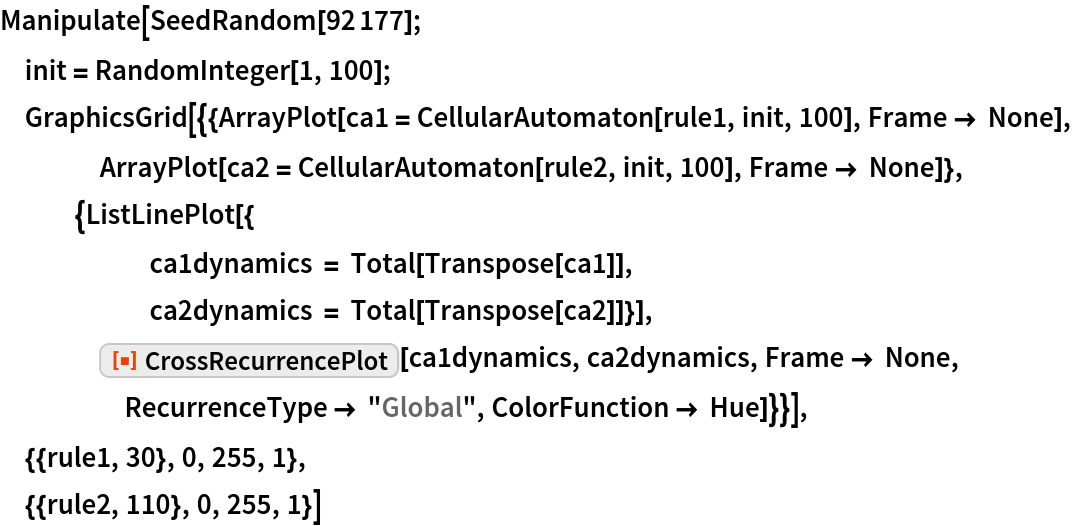Out=